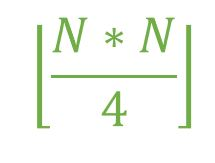# Maximum number of edges in Bipartite graph

Given an integer N which represents the number of Vertices. The Task is to find the maximum number of edges possible in a Bipartite graph of N vertices.

Bipartite Graph:

1. A Bipartite graph is one which is having 2 sets of vertices.
2. The set are such that the vertices in the same set will never share an edge between them.

Examples:

Input: N = 10
Output: 25
Both the sets will contain 5 vertices and every vertex of first set
will have an edge to every other vertex of the second set
i.e. total edges = 5 * 5 = 25

Input: N = 9
Output: 20

## Recommended: Please try your approach on {IDE} first, before moving on to the solution.

Approach: The number of edges will be maximum when every vertex of a given set has an edge to every other vertex of the other set i.e. edges = m * n where m and n are the number of edges in both the sets. in order to maximize the number of edges, m must be equal to or as close to n as possible. Hence, the maximum number of edges can be calculated with the formula,Below is the implementation of the above approach:

## C++

 `// C++ implementation of the approach ` `#include ` `using` `namespace` `std; ` ` `  `// Function to return the maximum number ` `// of edges possible in a Bipartite ` `// graph with N vertices ` `int` `maxEdges(``int` `N) ` `{ ` `    ``int` `edges = 0; ` ` `  `    ``edges = ``floor``((N * N) / 4); ` ` `  `    ``return` `edges; ` `} ` ` `  `// Driver code ` `int` `main() ` `{ ` `    ``int` `N = 5; ` `    ``cout << maxEdges(N); ` ` `  `    ``return` `0; ` `} `

## Java

 `// Java implementation of the approach ` ` `  `class` `GFG { ` ` `  `    ``// Function to return the maximum number ` `    ``// of edges possible in a Bipartite ` `    ``// graph with N vertices ` `    ``public` `static` `double` `maxEdges(``double` `N) ` `    ``{ ` `        ``double` `edges = ``0``; ` ` `  `        ``edges = Math.floor((N * N) / ``4``); ` ` `  `        ``return` `edges; ` `    ``} ` ` `  `    ``// Driver code ` `    ``public` `static` `void` `main(String[] args) ` `    ``{ ` `        ``double` `N = ``5``; ` `        ``System.out.println(maxEdges(N)); ` `    ``} ` `} ` ` `  `// This code is contributed by Naman_Garg. `

## Python3

 `# Python3 implementation of the approach  ` ` `  `# Function to return the maximum number  ` `# of edges possible in a Bipartite  ` `# graph with N vertices  ` `def` `maxEdges(N) :  ` ` `  `    ``edges ``=` `0``;  ` ` `  `    ``edges ``=` `(N ``*` `N) ``/``/` `4``;  ` ` `  `    ``return` `edges;  ` ` `  `# Driver code  ` `if` `__name__ ``=``=` `"__main__"` `: ` `     `  `    ``N ``=` `5``;  ` `    ``print``(maxEdges(N));  ` ` `  `# This code is contributed by AnkitRai01 `

## C#

 `// C# implementation of the approach ` `using` `System; ` ` `  `class` `GFG { ` ` `  `    ``// Function to return the maximum number ` `    ``// of edges possible in a Bipartite ` `    ``// graph with N vertices ` `    ``static` `double` `maxEdges(``double` `N) ` `    ``{ ` `        ``double` `edges = 0; ` ` `  `        ``edges = Math.Floor((N * N) / 4); ` ` `  `        ``return` `edges; ` `    ``} ` ` `  `    ``// Driver code ` `    ``static` `public` `void` `Main() ` `    ``{ ` `        ``double` `N = 5; ` `        ``Console.WriteLine(maxEdges(N)); ` `    ``} ` `} ` ` `  `// This code is contributed by jit_t. `

## PHP

 ` `

Output:

```6
```

Attention reader! Don’t stop learning now. Get hold of all the important DSA concepts with the DSA Self Paced Course at a student-friendly price and become industry ready.

My Personal Notes arrow_drop_upCheck out this Author's contributed articles.

If you like GeeksforGeeks and would like to contribute, you can also write an article using contribute.geeksforgeeks.org or mail your article to contribute@geeksforgeeks.org. See your article appearing on the GeeksforGeeks main page and help other Geeks.

Please Improve this article if you find anything incorrect by clicking on the "Improve Article" button below.

Improved By : AnkitRai01, jit_t, Naman_Garg

Article Tags :
Practice Tags :

1

Please write to us at contribute@geeksforgeeks.org to report any issue with the above content.